Get inspired by the success stories of our students in IIT JAM MS, ISI  MStat, CMI MSc DS.  Learn More

# The Unique Decomposition | ISI MStat 2015 PSB Problem 3The solution plays with Eigen values and vectors to solve this cute and easy problem in Linear Algebra from the ISI MStat 2015 problem 3.

## Problem

Let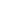be a real valued and symmetric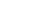matrix with entries such that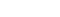and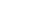.
(a) Prove that there exist non-zero column vectors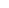and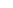such that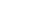and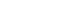.
(b) Prove that every vectorhas a unique decomposition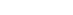where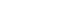and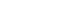.

This problem is from ISI MStat 2015 PSB ( Problem #3).

### Prerequisites

• Eigen values and Eigen vectors

## (a)

Let's say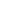is an eigenvalue of. Let's explore the possibilities of.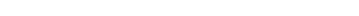. Since,is arbitrary, we get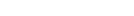.

Sinceis real symmetric, it has real eigenvalues, and the possibilities are 1 and -1. Since,, there exists non-zero column vectorsandsuch that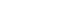and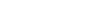.

## (b)

Supposehas two decompositions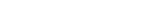whereandand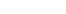and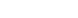.

Tberefore,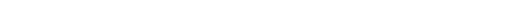.

But, we also have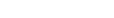. Thus, by adding and subtracting, we get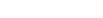.

The solution plays with Eigen values and vectors to solve this cute and easy problem in Linear Algebra from the ISI MStat 2015 problem 3.

## Problem

Letbe a real valued and symmetricmatrix with entries such thatand.
(a) Prove that there exist non-zero column vectorsandsuch thatand.
(b) Prove that every vectorhas a unique decompositionwhereand.

This problem is from ISI MStat 2015 PSB ( Problem #3).

### Prerequisites

• Eigen values and Eigen vectors

## (a)

Let's sayis an eigenvalue of. Let's explore the possibilities of.. Since,is arbitrary, we get.

Sinceis real symmetric, it has real eigenvalues, and the possibilities are 1 and -1. Since,, there exists non-zero column vectorsandsuch thatand.

## (b)

Supposehas two decompositionswhereandandand.

Tberefore,.

But, we also have. Thus, by adding and subtracting, we get.

This site uses Akismet to reduce spam. Learn how your comment data is processed.

### Knowledge Partner